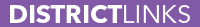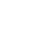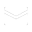Home About Us Admissions Departments College & Career Community Engagement Center for Art & LearningDepartments Academics Arts Health Services School Counseling Paideia Student Services H.R.Ladenheim Library Course Catalog

 Department Course Catalog

7-8 Accelerated Math 7 (MTH121)

Credit Type: Mathematics
Prerequisites: Placement Assessment

Accelerated Math 7 prepares 7th grade students to enter Algebra 1 as 8th graders. The course covers the content in both Math 7 and Math 8, with an emphasis on the 8th grade material. The course embeds the Common Core Standards for Mathematical Practice as an integral component of lessons. Students should expect nightly homework assignments (approximately 30 minutes), major assessments (tests, projects, quizzes) every three weeks, and a final exam at the end of the year.

7-8 Algebra 1 (MTH078)

Credit Type: Mathematics
Prerequisites: Math 7 Accelerated OR Placement Assessment

In Algebra 1, students continue to build their understanding of the four representations of linear, quadratic, and exponential functions, data modeling, and manipulating algebraic expressions. They also develop proficiency with rational numbers and exponents. The course embeds the Common Core Standards for Mathematical Practice as an integral part of lessons. Students should expect nightly homework assignments (30-45 minutes), major assessments (tests, projects, quizzes) every three weeks, and a final exam at the end of the year.

7-8 Math 7 (MTH107)

Credit Type: Mathematics
Prerequisites: None

Students focus on number sense (integers, fractions, decimals, and percents), 2D/3D geometry, expressions and equations, ratios and proportions, and probability. The course embeds the Common Core Standards for Mathematical Practice as an integral part of lessons. Students should expect nightly homework (approximately 30 minutes), major assessments (tests, projects, quizzes) every three weeks, and a final exam at the end of the year.

7-8 Math 8 (MTH108)

Credit Type: Mathematics
Prerequisites: None

Students focus on equations and variables, transformational geometry, Pythagorean Theorem, systems of equations, problem solving, exponents and roots, and 3D geometry. The course embeds the Common Core Standards for Mathematical Practice as an integral part of lessons. Students should expect nightly homework (approximately 30 minutes), major assessments (tests, projects, quizzes) every three weeks, and a final exam at the end of the year.

7-8 Math Skills 1

Credit Type: Mathematics

Math Skills covers basic mathematical concepts and operations that prepare students for more advanced mathematics courses. Curriculum is tailored to students' individual needs.

7-8 Math Skills 2

Credit Type: Mathematics

Math Skills covers basic mathematical concepts and operations that prepare students for more advanced mathematics courses. Curriculum is tailored to students' individual needs.

Academic Teaching Assistant in Middle School or High School Classes

Credit Type: School Service
Prerequisites: Audition and/or Interview

High school students may arrange to assist in a middle school academic class in which they have significant experience with the discipline, or a high school class in which they have already earned credit. This is an opportunity to earn school service credit, but NOT academic credit. TAs are expected to check in with the cooperating teacher regularly, participate fully in each class, model class expectations, assist in supporting students and the teacher whenever possible, and engage in pre-planning if taking a lead role as teacher. Prior teacher permission is required.

Algebra 1 (MTH109)

Credit Type: Mathematics
Prerequisites: Math 8 OR Placement Assessment

In Algebra 1, students continue to build their understanding of the four representations of linear, quadratic, and exponential functions, data modeling, and manipulating algebraic expressions. They also develop proficiency with rational numbers and exponents. The course embeds the Common Core Standards for Mathematical Practice as an integral part of lessons. Students should expect nightly homework assignments (30-45 minutes), major assessments (tests, projects, quizzes) every three weeks, and a final exam at the end of the year.

Algebra 1a

Credit Type: Mathematics

Algebra 1a and Algebra 1b comprise a two-year sequence that covers the same content as the standard Algebra 1 course.

Algebra 1b

Credit Type: Mathematics
Prerequisites: Algebra 1a

Algebra 1a and Algebra 1b comprise a two-year sequence that covers the same content as the standard Algebra 1 course.

Algebra 2 (MTH111)

Credit Type: Mathematics
Prerequisites: Geometry or co-enrolled in Geometry

Algebra 2 applies and extends what students have learned in previous courses through exploration of function transformations, equivalence, inverse equations, trigonometric functions, and the role of randomness and the normal distribution in making statistical conclusions. The course embeds the Common Core Standards for Mathematical Practice as an integral part of lessons. Students should expect daily homework assignments (about an hour per class), and tests approximately every three weeks. Students who elect to take Algebra 2 concurrently with Geometry must have a final grade of 3.2 in Algebra 1 (or similar from a prior school).

Computer Science (MTH114)

Credit Type: Mathematics
Prerequisites: Algebra 2 or Administrator Recommendation

This course is an introduction to writing computer code. Students learn the basics of a programming language, how to structure code to accomplish certain tasks, how to debug code that isn't working, and how to design algorithmic structures that enhance the efficiency and aesthetic quality of a program.

Financial Math (MTH119)

Credit Type: Mathematics
Prerequisites: Algebra 2 or Administrator Recommendation

This course is intended for seniors who do not plan to go into math-related fields, or higher education study. It focuses on topics related to personal finance including taxes, insurance, banking, borrowing, investing, and budgeting.

Fundamentals of Geometry

Credit Type: Mathematics

Fundamentals of Geometry addresses the same course objectives as the standard Geometry course, however the content is adjusted to assess these same objectives differently. Students delve more deeply into core concepts like Geometry on the Coordinate Grid, Area and Volume, Trigonometry, and Probability Modeling, but do so at a slower pace. In order to make up this extra commitment of time, some aspects of the standard Geometry curriculum are de-emphasized or omitted. Students should expect weekly homework and regular assessment via quizzes, projects, portfolio pieces, and unit tests.

Geometry (MTH110)

Credit Type: Mathematics
Prerequisites: Algebra 1 or co-enrolled in Algebra 1

In Geometry, students prove and apply geometric theorems about lines, triangles, quadrilaterals, circles, and three-dimensional solids. They also explore patterns and consistencies in the transformation of figures on the Cartesian plane. Finally, students develop models to investigate independent and conditional probabilities. The course embeds the Common Core Standards for Mathematical Practice as an integral part of lessons. Students should expect nightly homework (30-45 minutes), major assessments (tests, projects, quizzes) every three weeks, and a final exam at the end of the year. Students who elect to take Algebra 1 and Geometry concurrently must be recommended by their Math 8 teacher.

HIgh School Math Skills 1

Credit Type: Mathematics

High School Math Skills (formerly "Foundations of Math") is designed to build on students' skills and understanding of foundational pre-algebraic concepts. Emphasis is placed on mastering skills while also learning how to think about, and reason through, a variety of problems. The curriculum and class materials will be differentiated to meet individual student needs as they develop the content base required to succeed in an Algebra 1 course.

HIgh School Math Skills 2

Credit Type: Mathematics

High School Math Skills (formerly "Foundations of Math") is designed to build on students' skills and understanding of foundational pre-algebraic concepts. Emphasis is placed on mastering skills while also learning how to think about, and reason through, a variety of problems. The curriculum and class materials will be differentiated to meet individual student needs as they develop the content base required to succeed in an Algebra 1 course.

Honors AB Calculus (MTH126)

Credit Type: Mathematics
Prerequisites: Pre-Calculus or Placement Assessment

This is an advanced "Honors" level course in single-variable calculus that includes techniques and applications of the derivative, techniques and applications of the definite integral, and the Fundamental Theorem of Calculus. It is equivalent to at least a semester of calculus at most colleges and universities. Algebraic, numerical, and graphical representations are emphasized throughout the course. Students will have enough exposure to Calculus to take the AP Calculus AB exam with independent studying required. Students should expect to receive nightly homework, quizzes every few weeks, and major assessments each unit.

Introduction to Programming and Game Design (MTH117)

Credit Type: Mathematics
Prerequisites: Algebra 2 or Administrator Recommendation

Use the Unity 3D game engine as a platform to learn the C# programming language. Through the development of several simple games, students explore the power of programming as well as the artistic expression that Unity makes accessible to everyone. The focus will be on the scripting side of Unity and not on the creation of 2D and 3D art assets.

Pre-calculus (MTH103)

Credit Type: Mathematics
Prerequisites: Algebra 2

Pre-calculus is primarily a survey of the families of functions that mathematicians utilize in trying to solve problems and make sense of the world. We examine how these functions behave, how we can manipulate them, and how we can use them to model the real world in order to derive meaningful and useful information. The course embeds the Common Core Standards for Mathematical Practice as an integral part of lessons. Students should expect nightly homework assignments (about an hour per class), and tests approximately every three weeks.

Statistics (MTH115)